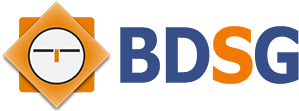# Mystery Case Files: Escape from Ravenhearst WalkthroughMystery Case Files: Escape from Ravenhearst Walkthrough
* OS: Windows 7 / Vista / XP
* CPU: Pentium 3 1.0 GHz or HigherMystery Case Files: Escape from Ravenhearst Walkthrough
* OS: Windows 7 / Vista / XP
* CPU: Pentium 3 1.0 GHz or Higher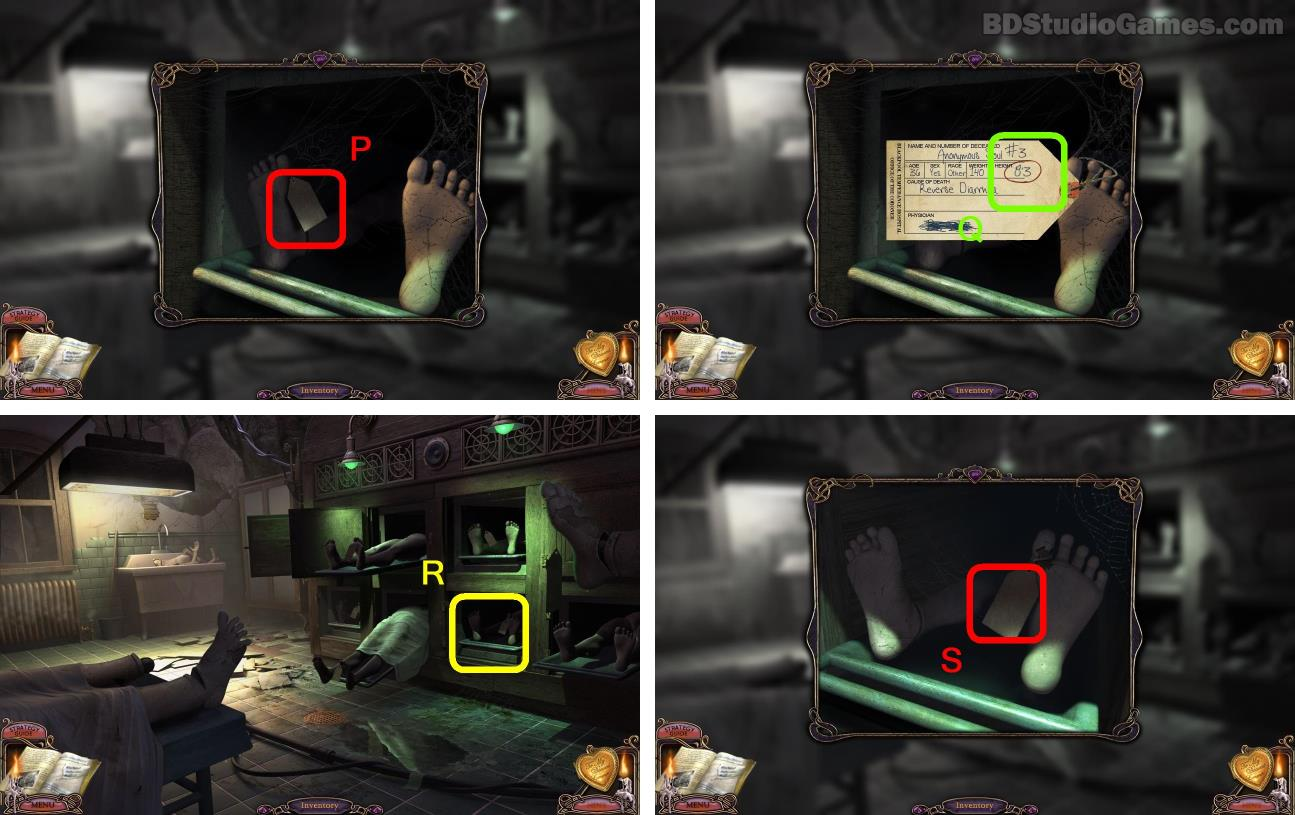Examine here. (P)
Write down this random number(CODE 6). (Q)
Examine here. (R)
Examine here. (S)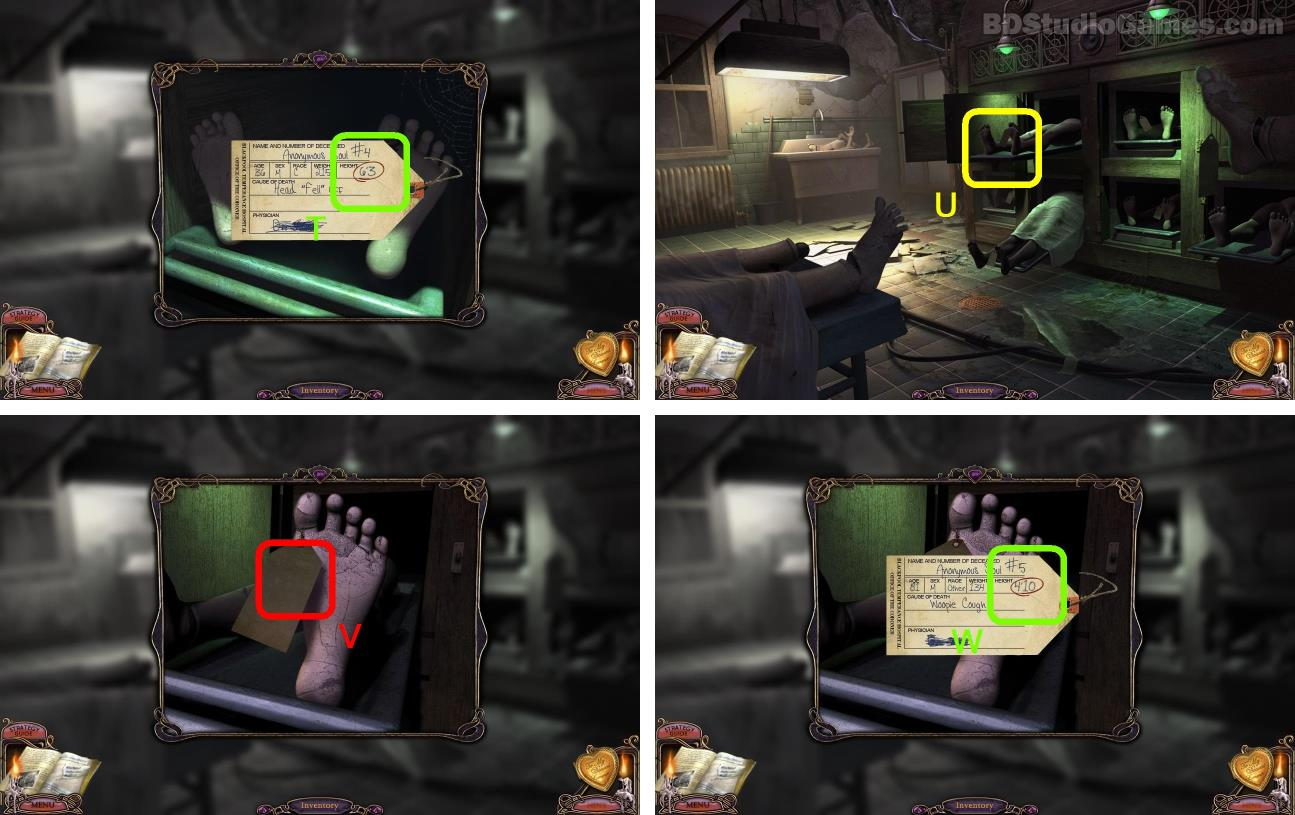Write down this random number(CODE 7). (T)
Examine here. (U)
Examine here. (V)
Write down this random number(CODE 8). (W)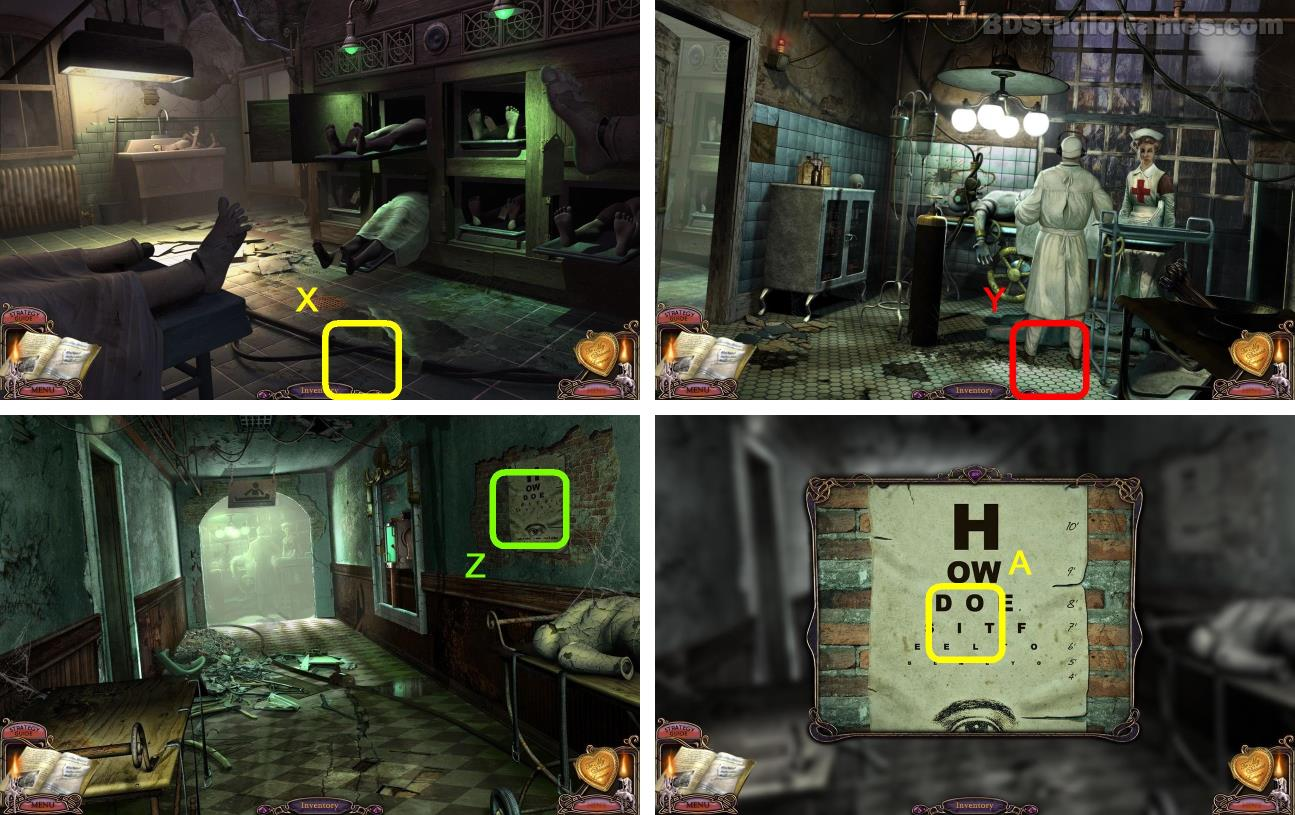Go Here. (X)
Go Here. (Y)
Examine here. (Z)
Use (CODE 4, 5, 6, 7, 8) solve the puzzle. For example, (CODE 4) is 7|4, find 7 on the eye chart right colume, then find the 4th letter on that row, this letter is F. Decrypt all (CODE 4, 5, 6, 7, 8) to letters. If the letter is too small to view, you should use your MAGNIFYING GLASS. (A)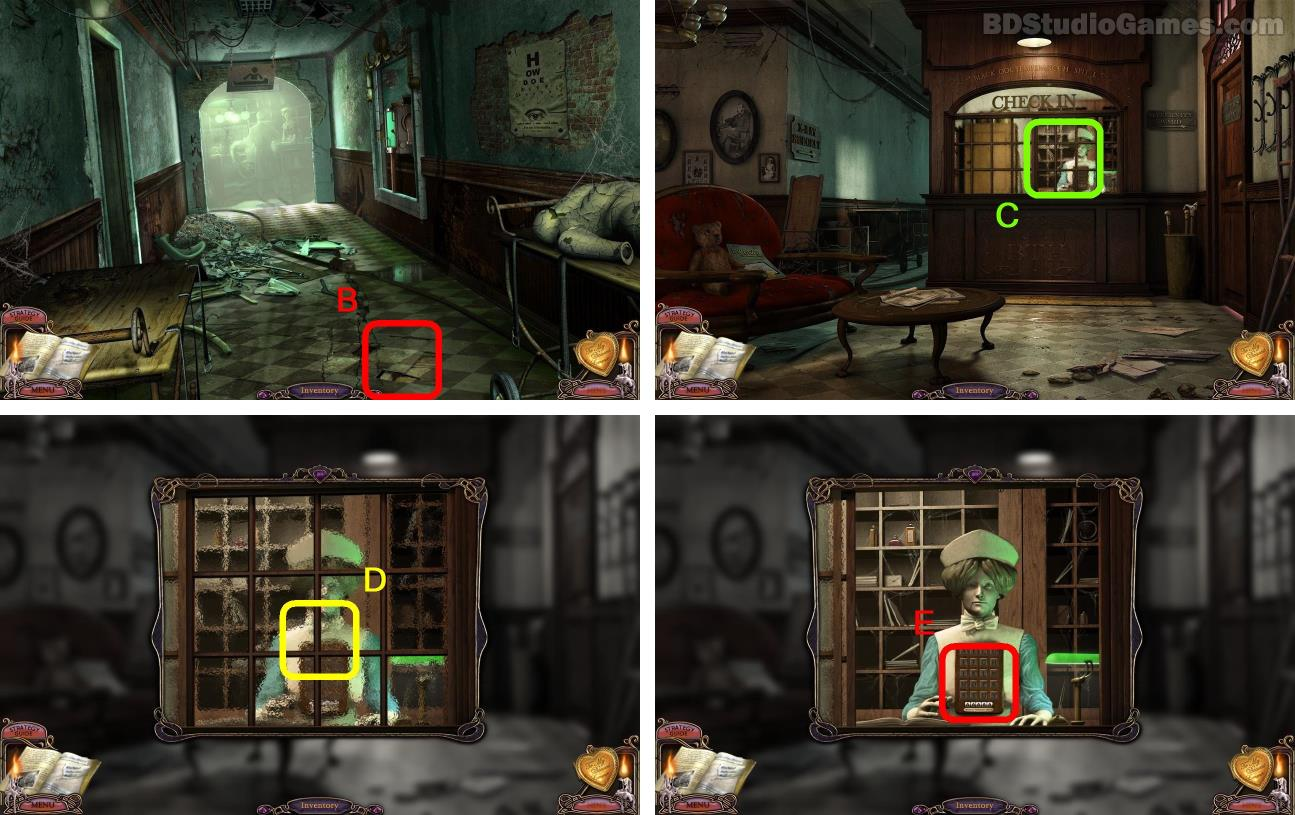Go Here. (B)
Examine here. (C)
Examine here. (E)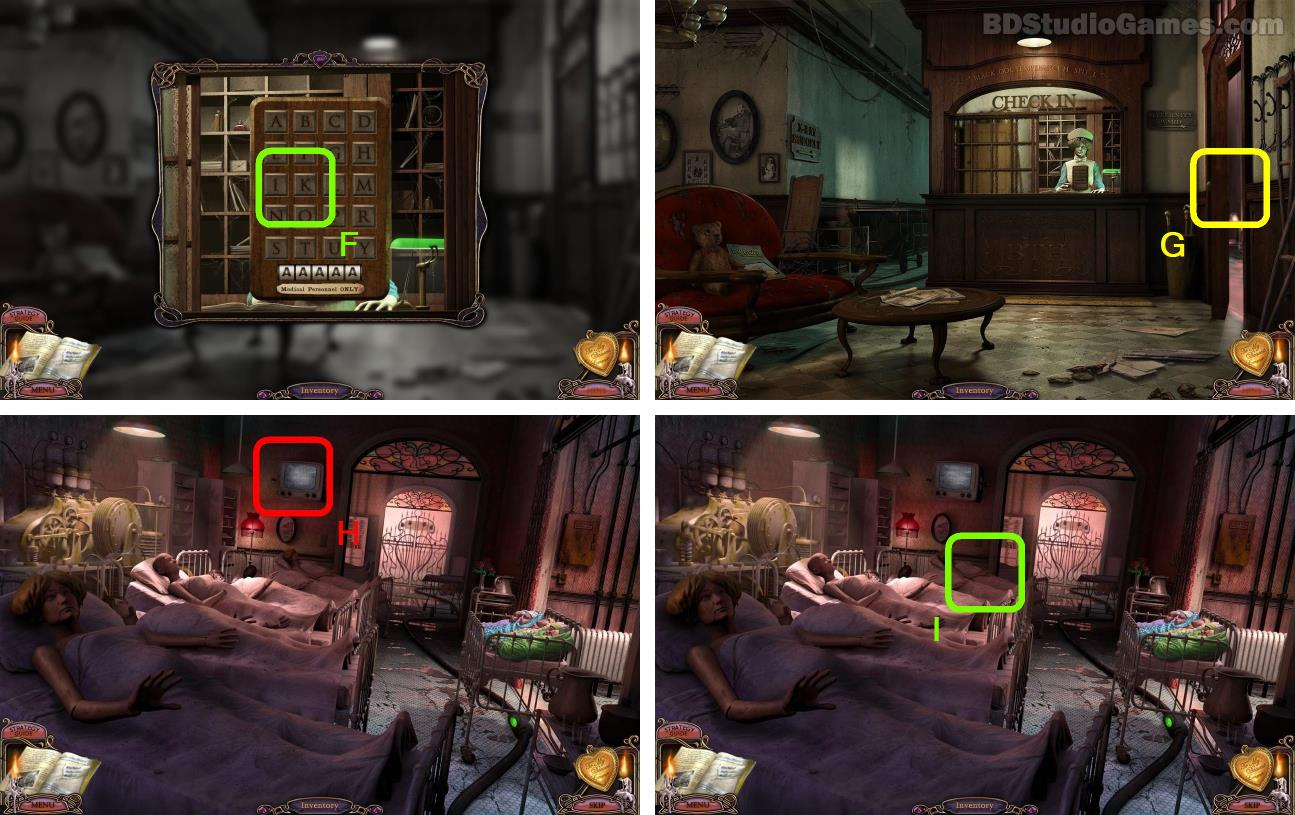Use your decrypt letters solve the puzzle. (F)
Go Here. (G)
Examine here. (H)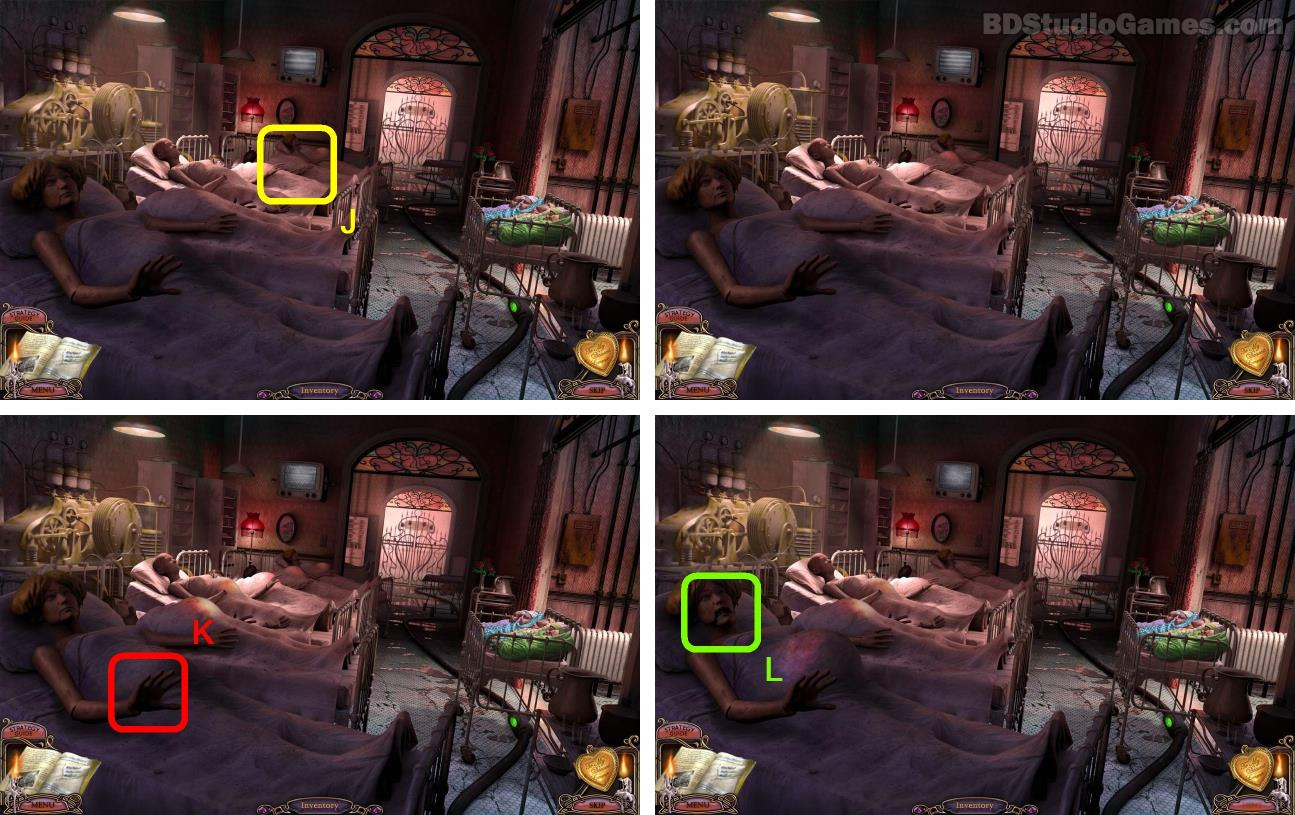To solve the puzzle, click on the stomachs in this order (A is the first lady on the left and E is the next to last): E x 2, D X 2, C x 2, B x 2, A x 2. (J)
Click the hand. (K)
Examine here. (L)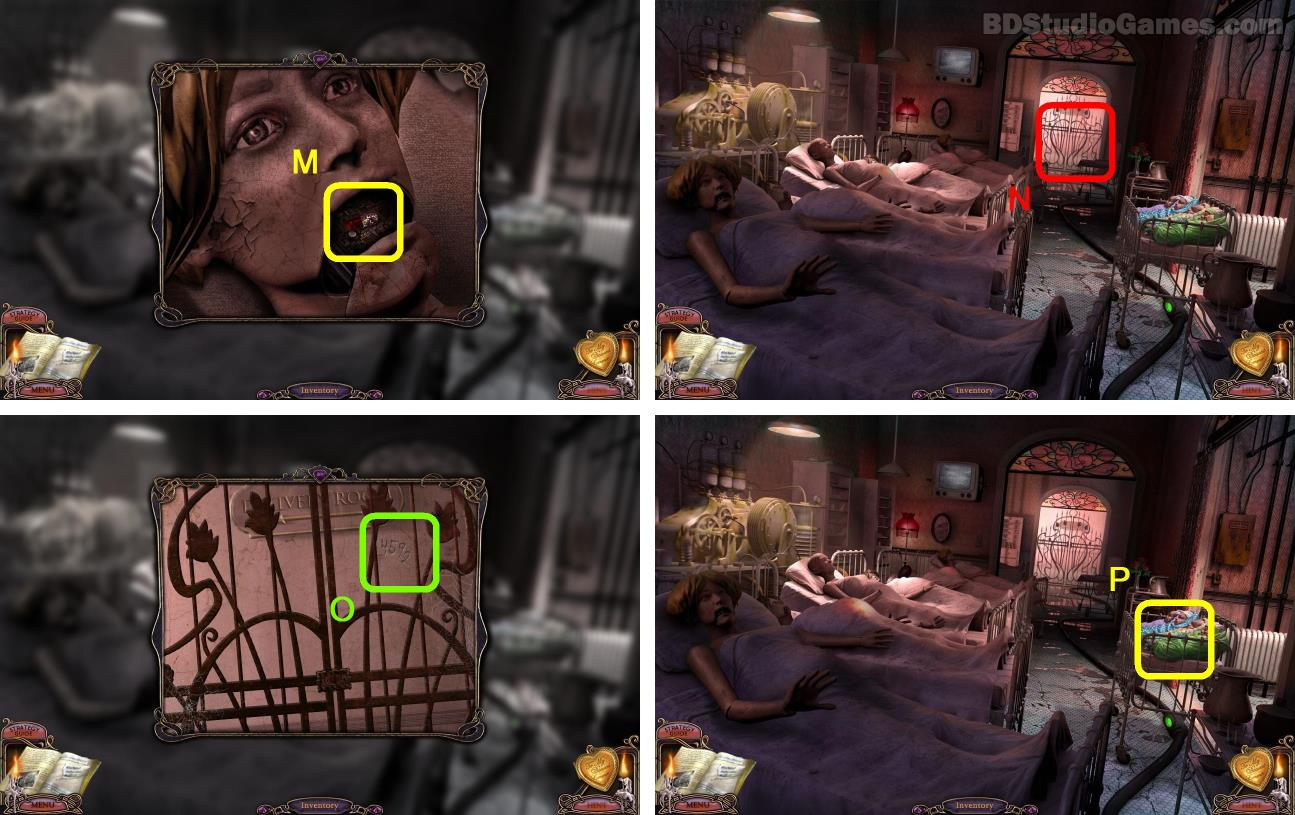Take it. (M)
Examine here. (N)
Write down this number 4592. (O)
Examine here. (P)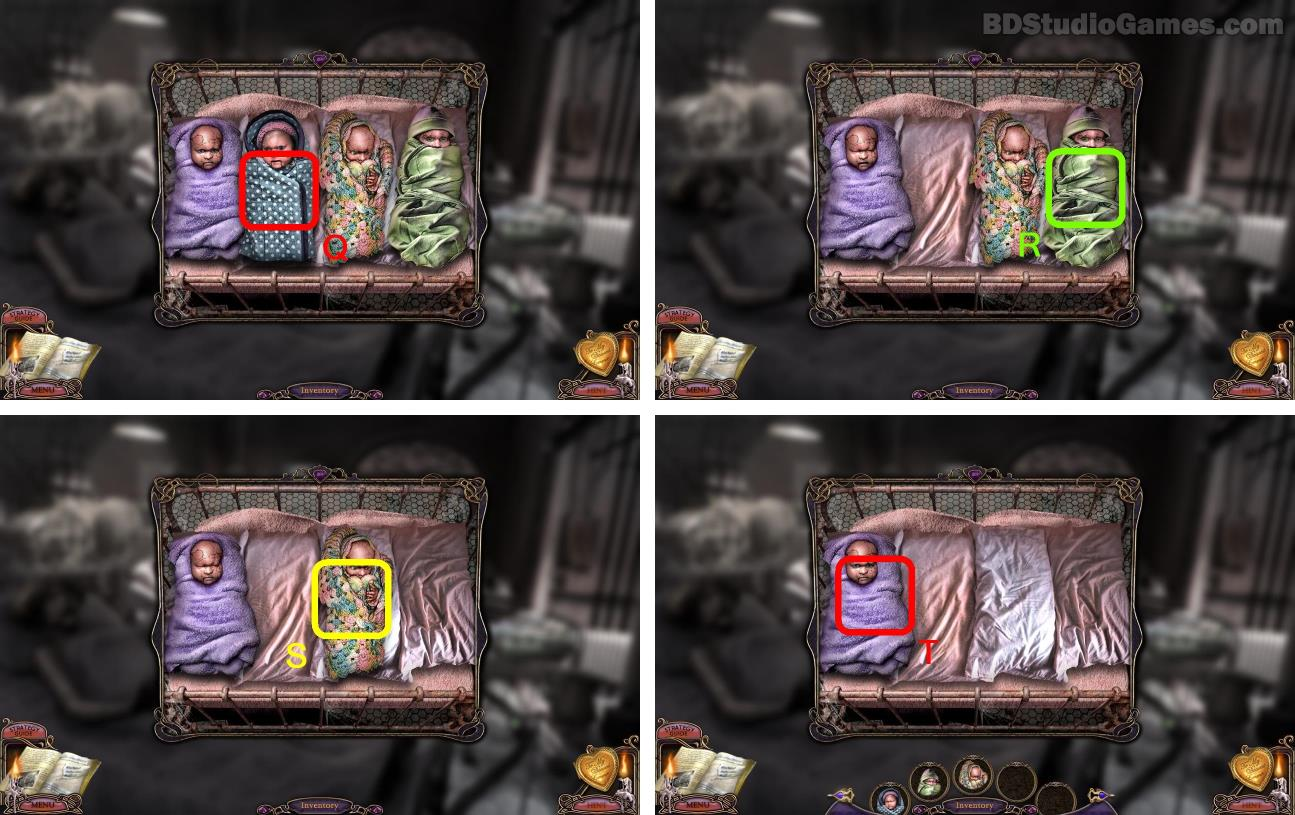Take it. (Q)
Take it. (R)
Take it. (S)
Take it. (T)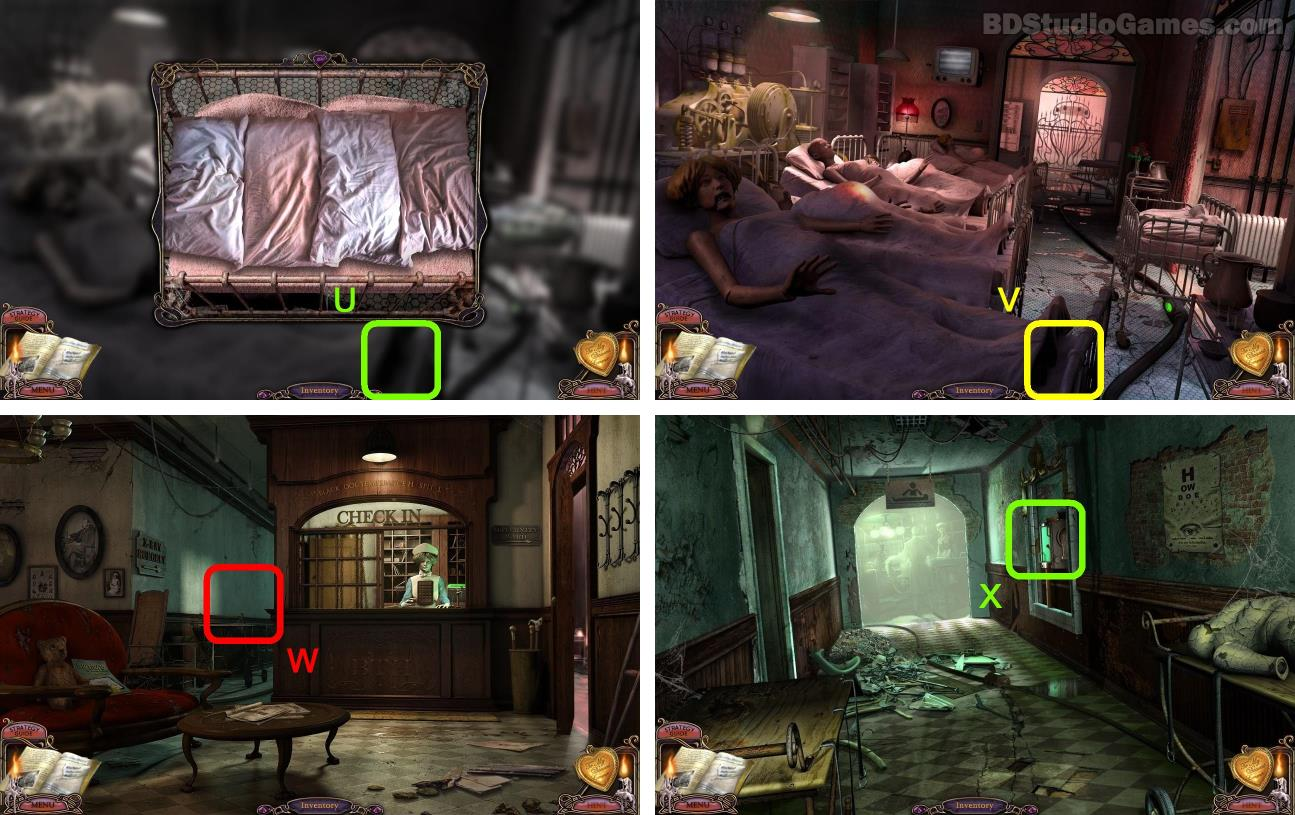Go Here. (U)
Go Here. (V)
Go Here. (W)
Examine here. (X)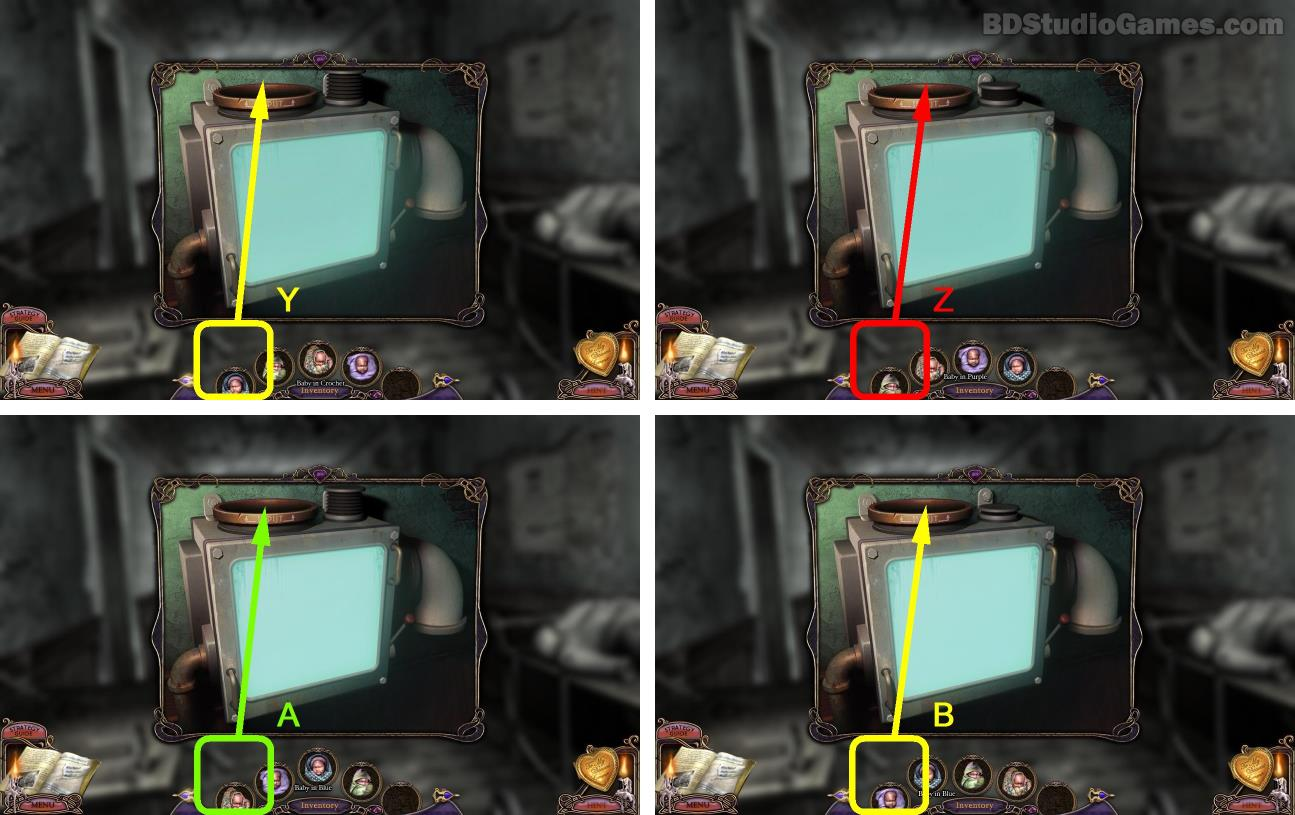Use object on here. Blue Baby. (Y)
Use object on here. Green Baby. (Z)
Use object on here. Crochet Baby. (A)
Use object on here. Purple Baby. (B)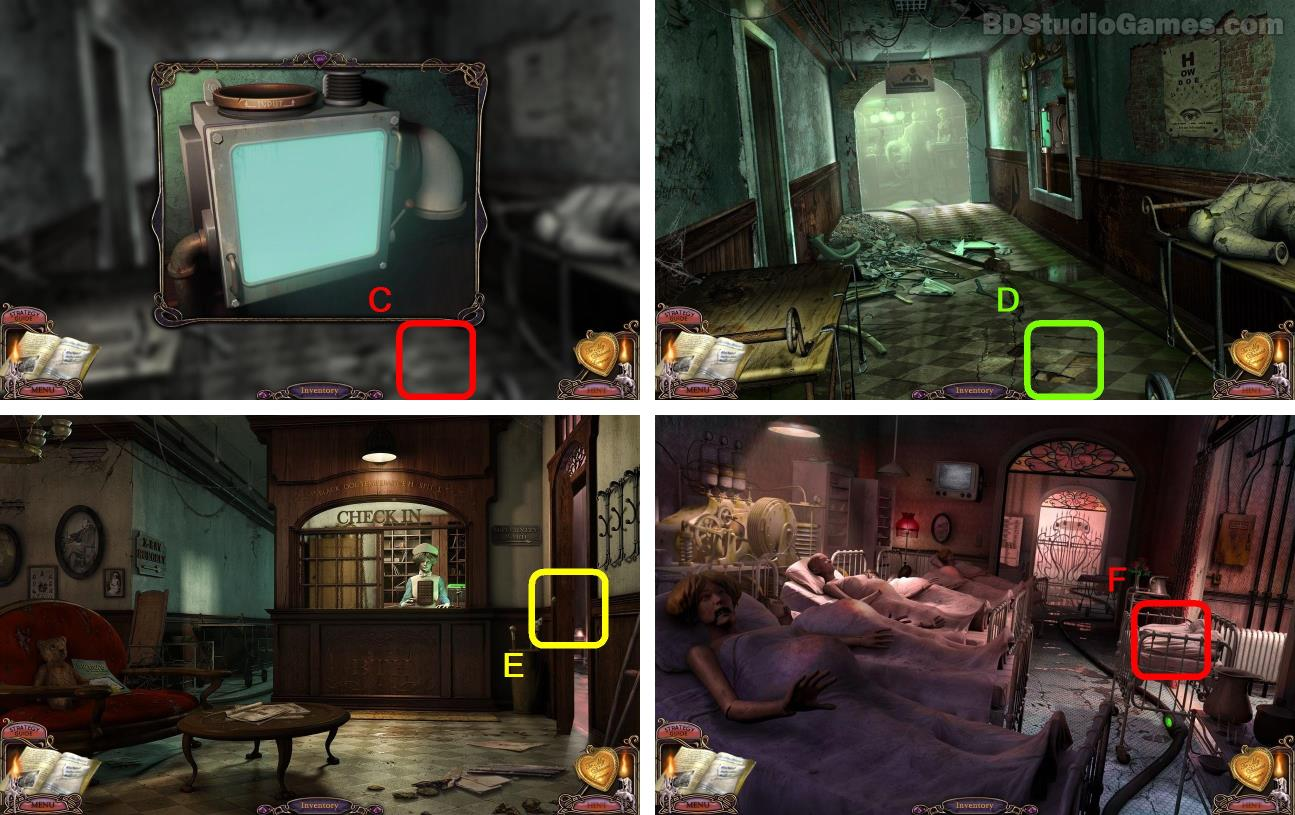Go Here. (C)
Go Here. (D)
Go Here. (E)
Examine here. (F)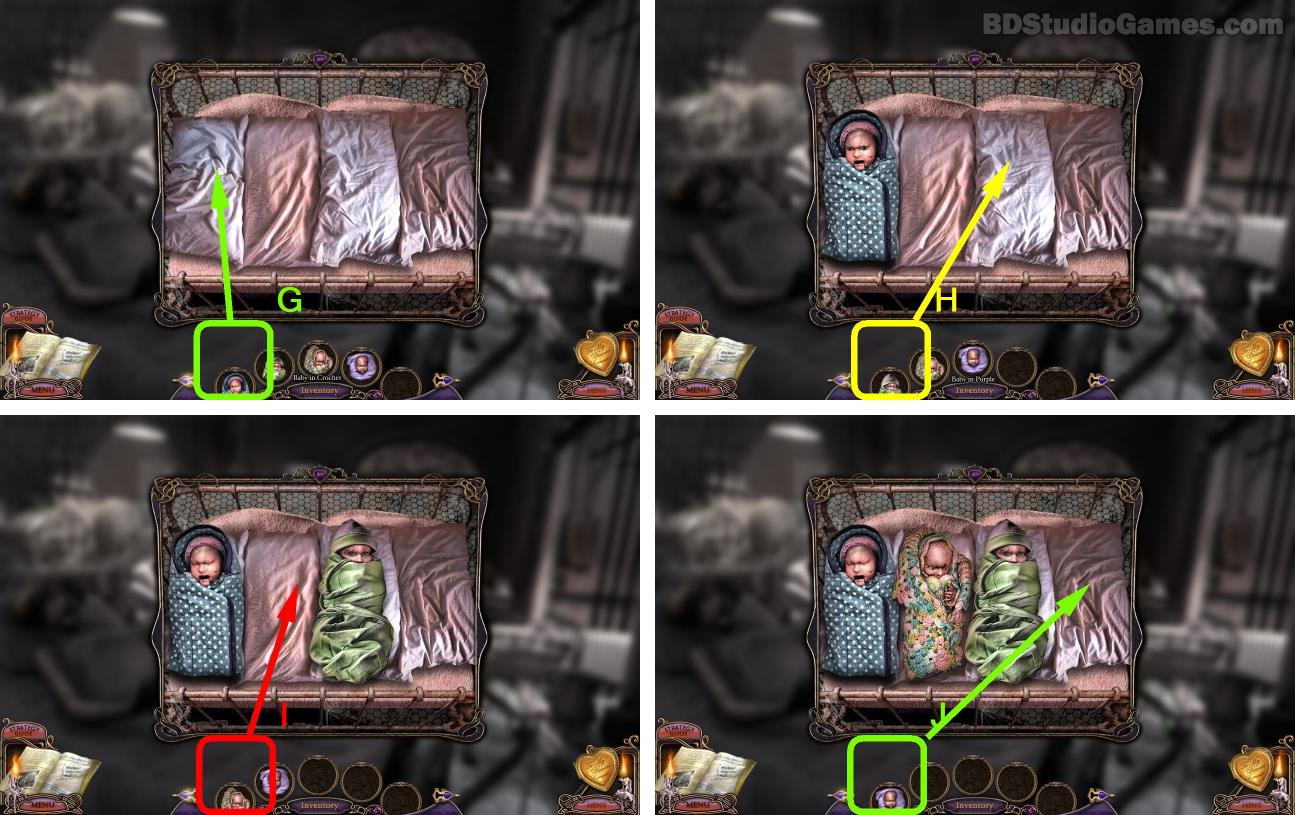Put blue baby to first position (G)
Put green baby to second position (H)
Put crochet baby to third position (I)
Put purple baby to 4th position (J)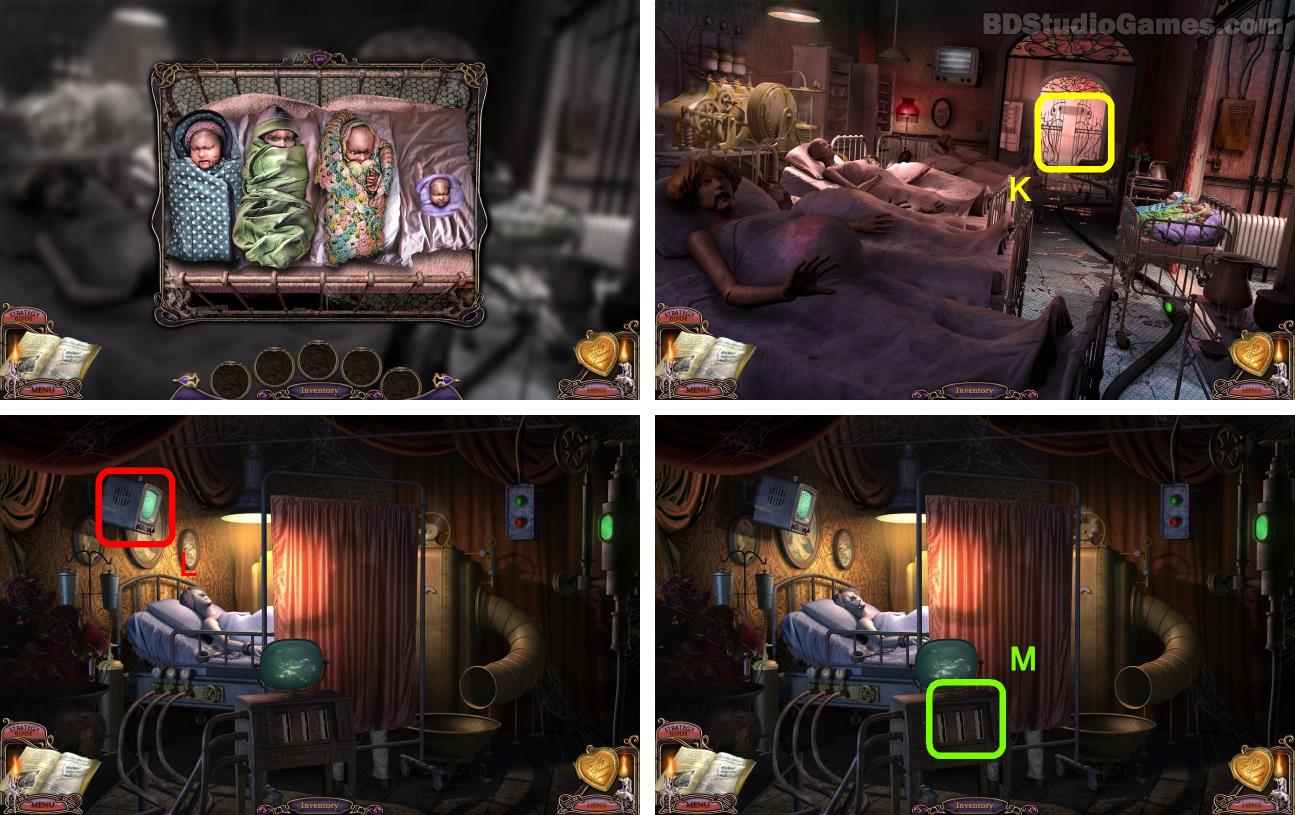Go Here. (K)
Examine here. (L)
Examine here. (M)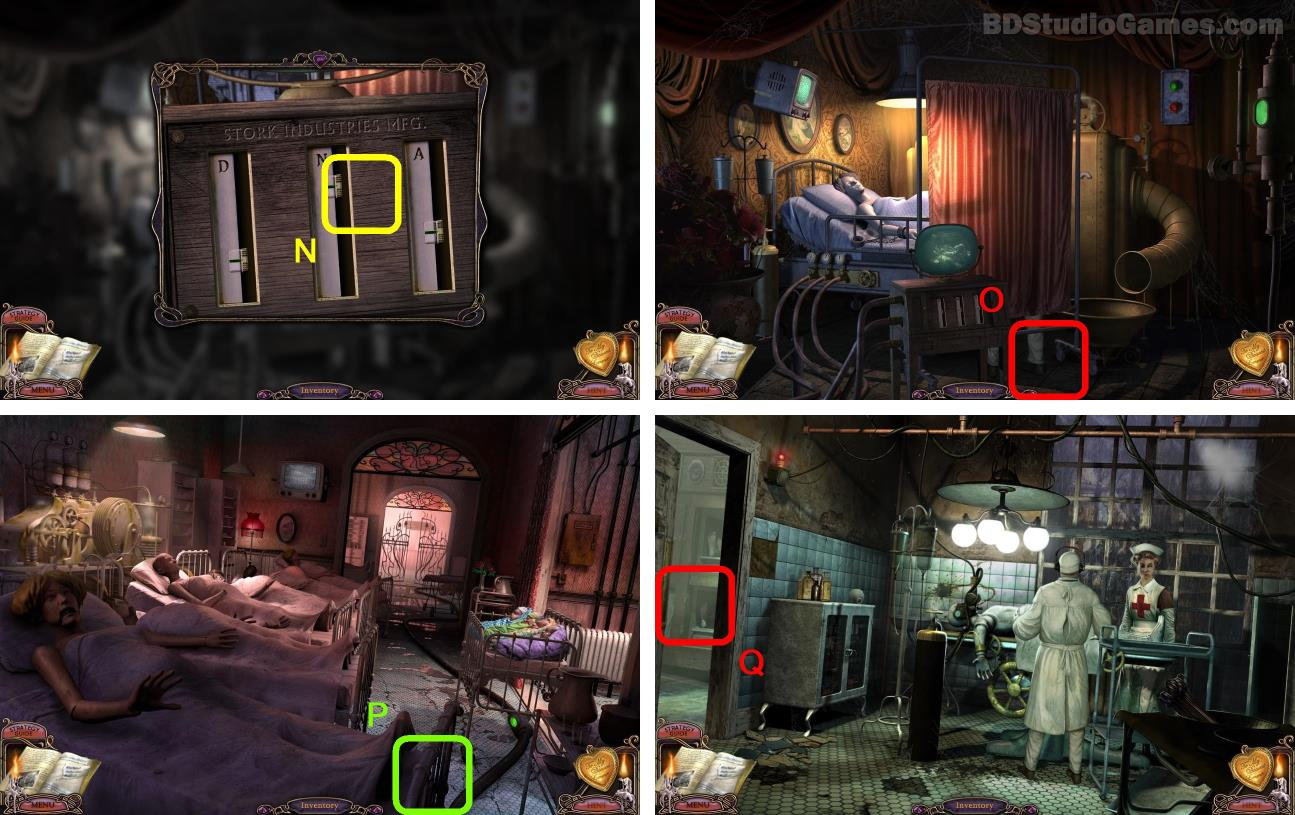Go Here. (O)
Go Here. (P)
Come to this place.
Go Here. (Q)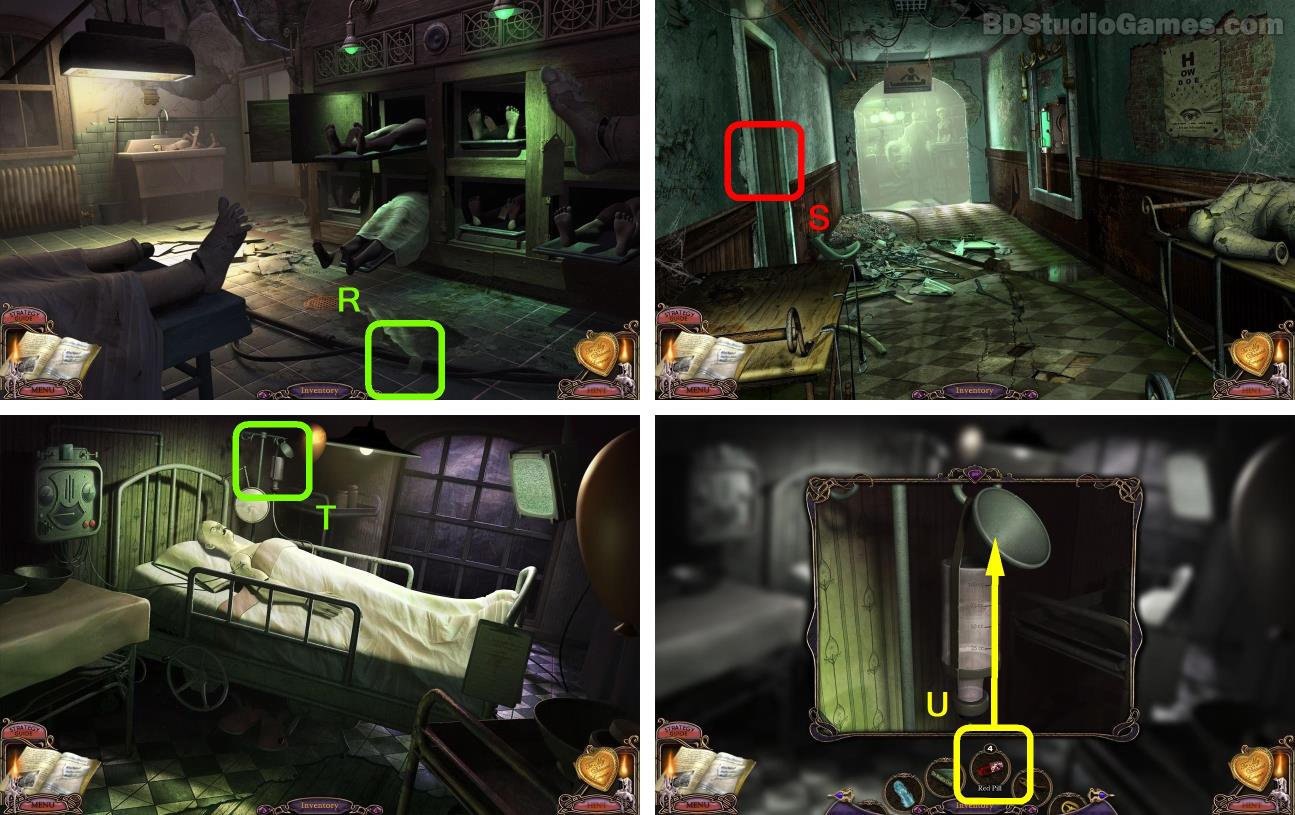Go Here. (R)
Come to this place.
Go Here. (S)
Examine here. (T)
Use object on here. (U)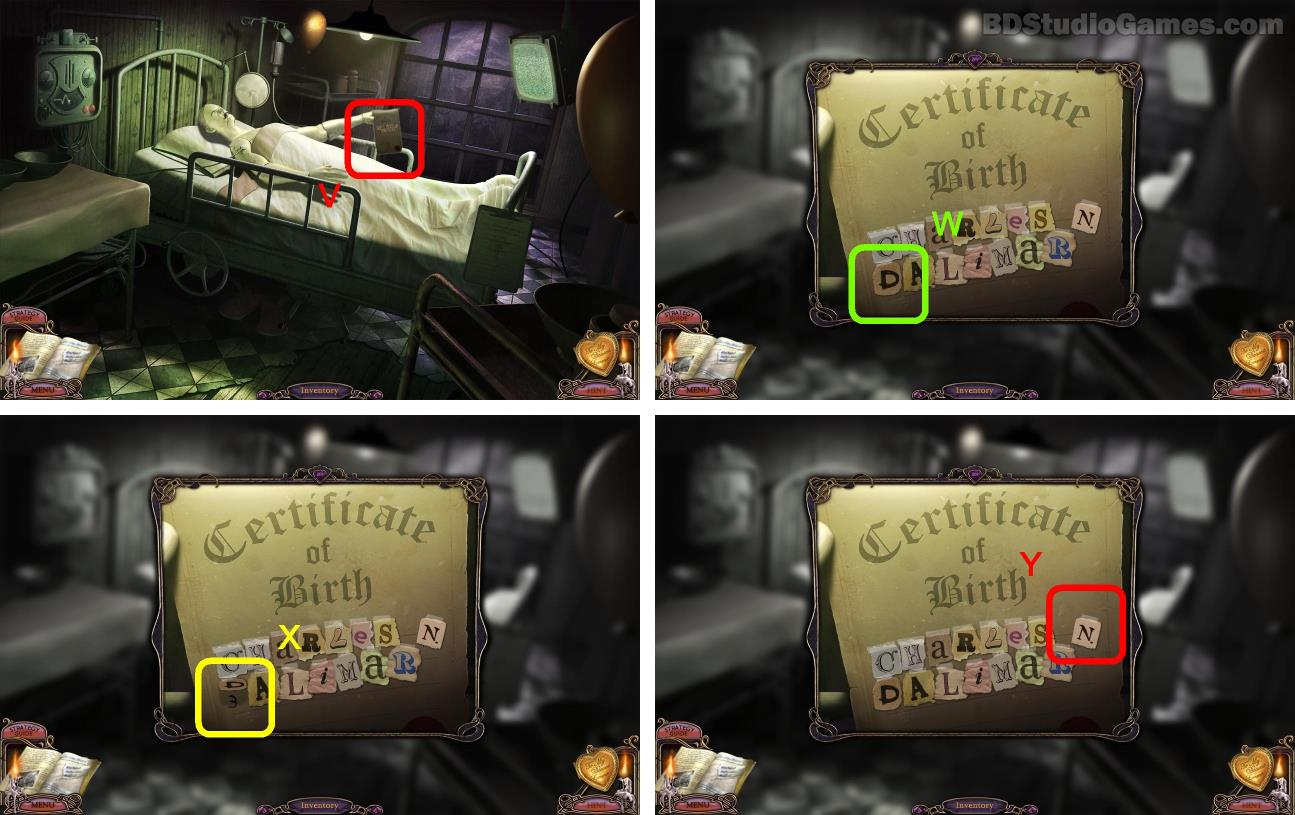Examine here. (V)
Click the letter D. (W)
Write down the number behind letter D, this number is random (CODE 9). (X)
Click the letter N. (Y)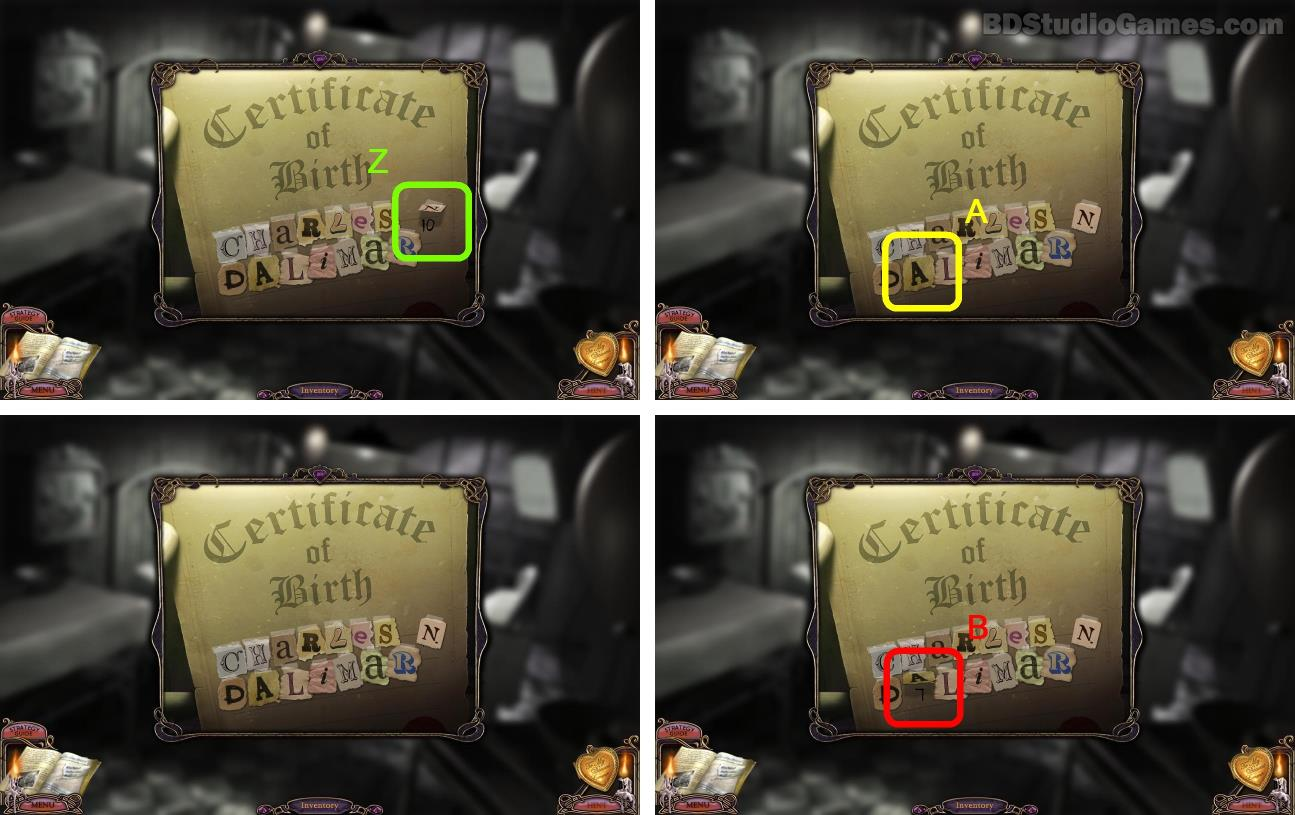Write down the number behind letter N, this number is random (CODE 10). (Z)
Click the letter A. (A)
Write down the number behind letter A, this number is random (CODE 11). (B)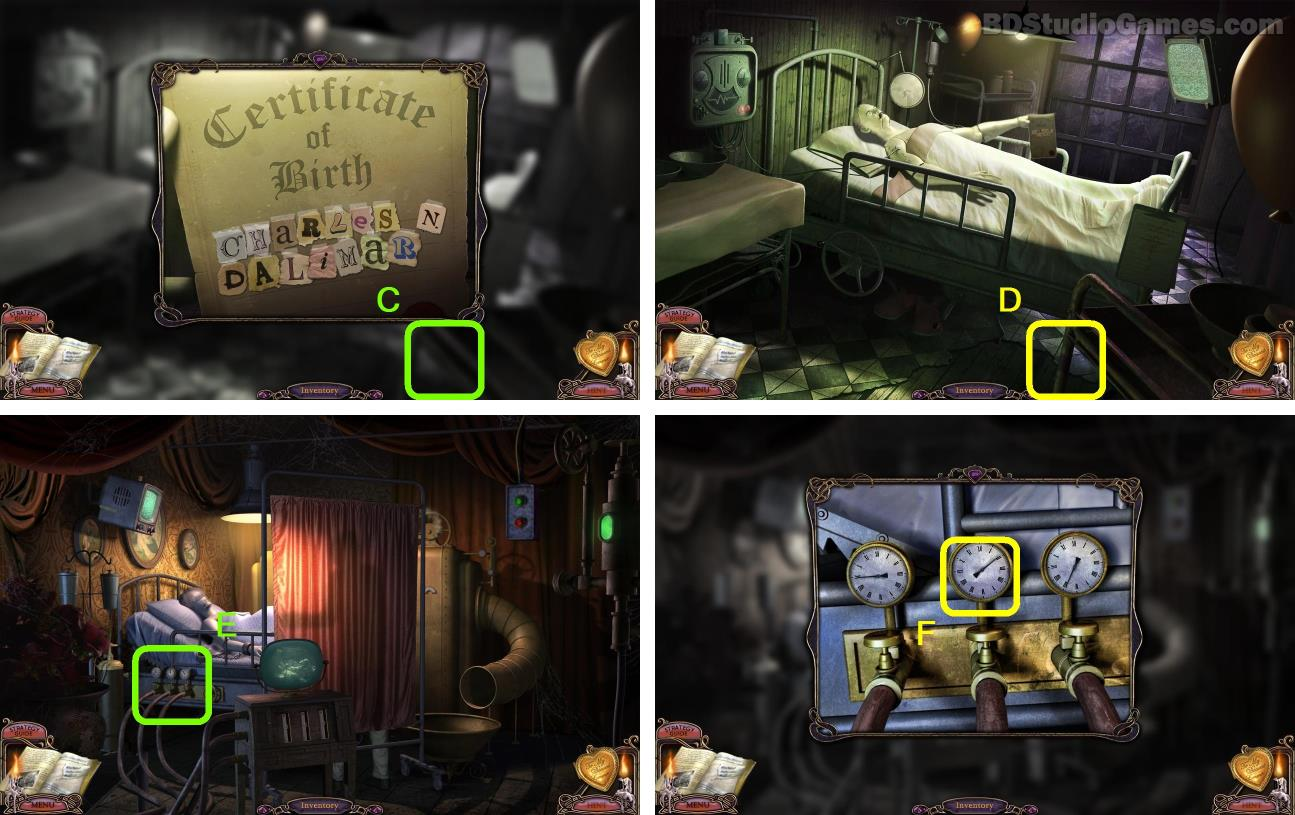Go Here. (D)
Come to this place.
Examine here. (E)
Examine here. (F)Examine here. (G)
Use the (CODE 9, 10, 11) solve the puzzle. CODE 9 - left slider, CODE 10 - middle slider, CODE 11 - right slider. Put the slider to top refer to the minimum number, put the slider to bottom refer to the maximum number. (H)
Once the slider are in the right position, click here for a baby to fall into the basin. (J)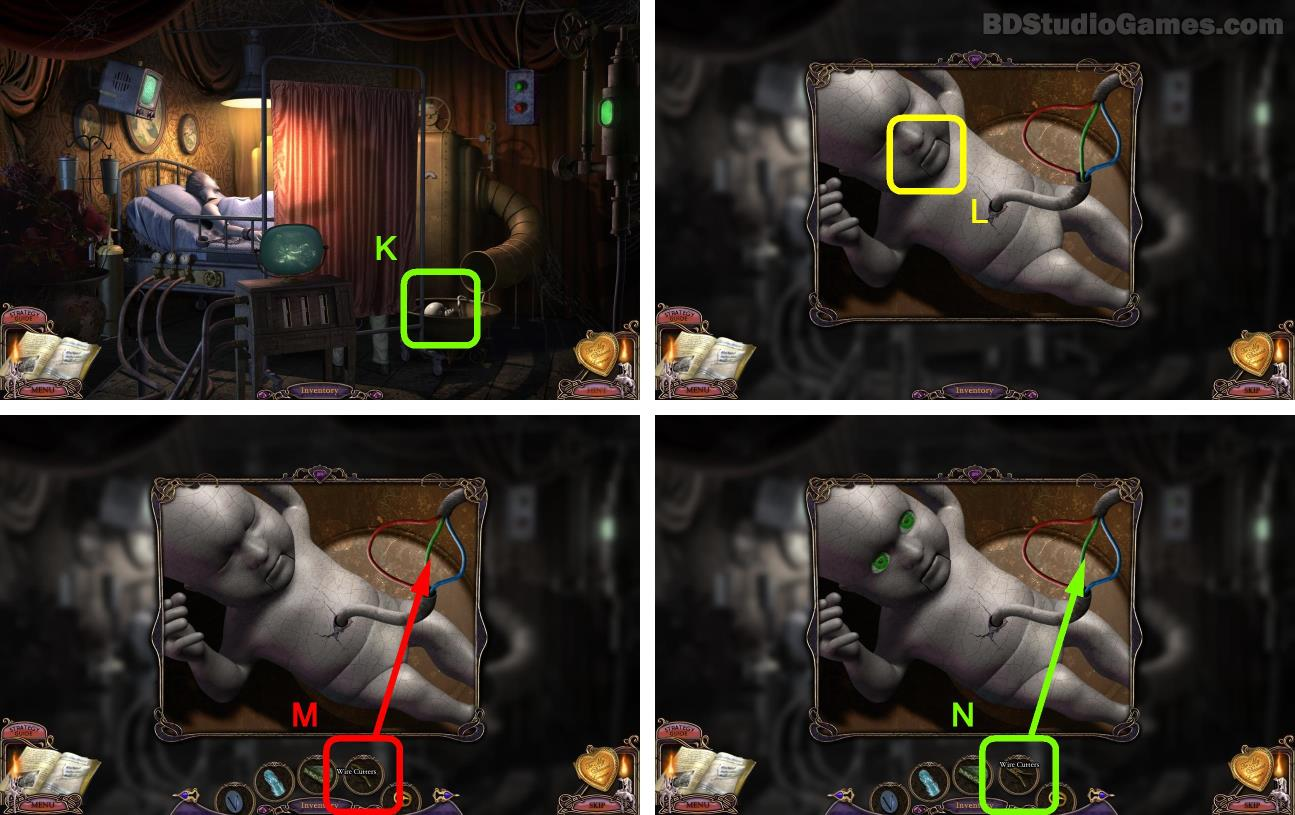Examine here. (K)
Click the mouth. (L)
Use WIRE CUTTERS to cut the green wire. (M)
You can do this only when the eye becomes green. (N)